# Algebraic K-theory

<]]>

<]]>
• ## Online References

Weibel's book project

Lectures notes of Schlichting

Weibel's K-theory handbook chapter: K0691

Short course by Levine

Gillet: K-theory and Intersection theory. Treats relation to Chow groups, coniveau filtration, Gersten's conjecture and more. Also the stable homotopy-theoretic viewpoint on algebraic K-theory, and K-theory as sheaf hypercohomology, and other basic things. Extremely nice article!

A survey by Arlettaz: Algebraic K-theory of rings from a topological viewpoint

Garkusha on a general construction recovering Karoubi-Villamayor and Quillen K-theory for rings.

The Development of algebraic K-theory before 1980, by Charles A. Weibel: K0343

Opérations sur la K-théorie algébrique et régulateurs via la théorie homotopique des schémas, by Joël Riou

Some notes by Rosenberg on relations with topology and analysis.

Something on the plus constuction by Berrick

Brown and Gersten: Algebraic K-theory as generalized sheaf cohomology (1973)

For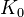and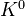of a variety, see de Jong's notes on Algebraic de Rham cohomology

Thomason's ICM talk 1990: The local to global principle in algebraic K-theory.

http://www.ncatlab.org/nlab/show/algebraic+K-theory

<]]>
• ## Paper References

See Kuku in Handbook of algebra Vol 4, for a survey of a number of different types of algebraic K-th including Volodin K-th.

Bass: Algebraic K-theory (1968) in K-th folder. Treats K0 and K1 or rings. Background on rings and projective modules. Reciprocity laws (ch VI and XIII). Finiteness thms for rings of arithmetic type (Ch X).

Read Quillen\'s original paper!! Here is a review/summary. See also the sequel by Grayson. There is also a survey article by Swan which seems to summarize much of Quillen\'s paper, possibly taking into account later developments.

Geisser in the K-theory handbook is excellent.

Grayson has a survey in the Motives volumes.

Thomason-Trobaugh: Higher algebraic K-theory of schemes and of derived categories. In the Grothendieck Festschrift, Vol III.

Milnor: Intorduction to algebraic K-theory (1971)

Rosenberg: Algebraic K-theory and its applications.

Soulé et al: Lectures on Arakelov Geometry: First chapter treats Chow groups, with product induced from isomorphism with K-groups. Good quick review of algebraic K-theory.

Many papers by Gillet, including stuff on Chern classes in a very general setting.

Grayson Hangzhou lectures in K-th folder: Brief intro to algebraic K-theory, very readable, with end remarks on motivic cohomology.

Handbook of K-th?

Friedlander's ICTP lectures in K-th folder: Very nice introduction to algebraic K-theory.

Levine Morelia lecture notes: Basic intro to algebraic K-theory of rings and schemes.

Manin: Lectures on the K-functor in algebraic geometry. In K-th folder. Among other things, he covers monoidal transformations and Riemann-Roch.

Milnor: Introduction to algebraic K-th (in K-th folder). Covers mainly K2, note that these notes came before Quillen's work.

Rosenberg in K-th folder: Basic intro to algebraic K-theory of rings: concrete approaches to K0, K1, K2, negative K-theory, plus and Q constructions, cyclic homology.

Srinivas: Algebraic K-th book, K-th folder. Comprehensive introduction to algebraic K-theory of rings and schemes. Advanced topics include the Merkurjev-Suslin thm and localization for singular varieties.

Weibel: The K-book. Looks like a very good introduction to algebraic K-theory. See his webpage for the latest version, I have a version from Sep 2011.

<]]>
• ## Definition

First define K-groups of an exact cat. Then the cat of locally free sheaves of finte rank give K-groups, and if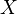is noetherian, the cat of coherent sheaves gives G-groups. Ifis regular, the two kinds of groups coincide.

From the definition, K-groups are contravariant functors from schemes to abelian groups. G-groups are contravariant for flat maps only.

For K-theory of rings, one brief introduction is Burgos-Gil, chapter 9. Using the Cartan-Serre theorem, can obtain information about the K-groups of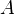by studying the homology of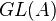("the homology of an H-space is a Hopf algebra").

The 5 papers by Neeman on K-theory of triangulated categories: K0507, K0508, K0509, K0510, K0511. Perhaps the K-theory handbook is a better source for this material.

On Voevodsky's algebraic K-theory spectrum BGL, by Ivan Panin, Konstantin Pimenov, and Oliver Roendigs: Under a certain normalization assumption we prove that the Voevodsky's spectrum BGL which represents algebraic K-theory is unique over the integers. Following an idea of Voevodsky, we equip the spectrum BGL with the structure of a commutative ring spectrum in the motivic stable homotopy category. Furthermore, we prove that under a certain normalization assumption this ring structure is unique over the integers. We pull this structure back to get a distinguished monoidal structure on BGL for an arbitrary Noetherian base scheme. K0838

http://mathoverflow.net/questions/1006/motivation-interpretation-for-quillens-q-construction

<]]>
• ## Properties

Charles A. Weibel, Homotopy algebraic $K$-theory (pp. 461--488) (1987, some proceedings)

http://mathoverflow.net/questions/984/algebraic-k-theory-and-tensor-products

arXiv:1301.3815 Universality of K-Theory fra arXiv Front: math.AG av José Luis González, Kalle Karu We prove that graded K-theory is universal among oriented Borel-Moore homology theories with a multiplicative periodic formal group law.

<]]>
• ## Standard theorems

See this review for different views on the localisation sequence

<]]>

<]]>
• ## Connections to Number Theory

Kolster: K-theory and arithmetic (K-th folder). Notes on zeta values of rings of integers, algebraic K-groups, etale cohomology, Iwasawa theory, motivic cohomology, Vandiver's conjecture.

Interesting paper by Dwyer and Mitchell.

See all papers by Morrow for higher local fields and arithmetic surfaces. For example: arXiv:1211.1533 K-theory of one-dimensional rings via pro-excision fra arXiv Front: math.KT av Matthew Morrow This paper studies "pro-excision" for the K-theory of one-dimensional (usually semi-local) rings and its various applications. In particular, we prove Geller's conjecture for equal characteristic rings over a perfect field of finite characteristic, give the first results towards Geller's conjecture in mixed characteristic, and we establish various finiteness results for the K-groups of singularities (covering both orders in number fields and singular curves over finite fields).

http://mathoverflow.net/questions/10204/any-reason-why-k23z-has-order-65520

For K-theory of (rings of integers of) global fields, see Weibel's survey in the Handbook of K-theory. Here is something about nontorsion elements. Here is something about even K-groups of Q and relations to cyclotomic conjectures, by Banaszak and Gajda

arXiv:1002.2936 Splitting in the K-theory localization sequence of number fields from arXiv Front: math.KT by Luca Caputo Let p be a rational prime and let F be a number field. Then, for each i>0, there is a short exact localization sequence for K{2i}(F). If p is odd or F is nonexceptional, we find necessary and sufficient conditions for this exact sequence to split: these conditions involve coinvariants of twisted p-parts of the p-class groups of certain subfields of the fields F(\mu{p^n}) for n\in N. We also compare our conditions with the weaker condition WK^{et}_{2i}(F)=0 and give some example.

MR1760901 (2001i:11082) Bloch, Spencer J.(1-CHI) Higher regulators, algebraic $K$-theory, and zeta functions of elliptic curves.

arXiv:0910.4005 The extended Bloch group and algebraic K-theory from arXiv Front: math.KT by Christian K. Zickert We define an extended Bloch group for an arbitrary field F, and show that this group is canonically isomorphic to K3^ind(F) if F is a number field. This gives an explicit description of K3^ind(F) in terms of generators and relations. We give a concrete formula for the regulator, and derive concrete symbol expressions generating the torsion. As an application, we show that a hyperbolic 3-manifold with finite volume and invariant trace field k has a fundamental class in K_3^ind(k) tensor Z[1/2].

arXiv:1101.5477 On special elements in higher algebraic K-theory and the Lichtenbaum-Gross Conjecture from arXiv Front: math.KT by David Burns, Herbert Gangl, Rob de Jeu We conjecture the existence of special elements in odd degree higher algebraic K-groups of number fields that are related in a precise way to the values at strictly negative integers of the derivatives of Artin L-functions of finite dimensional complex representations. We prove this conjecture in certain important cases and also provide other evidence (both theoretical and numerical) in its support.

arXiv:1208.2137 Wild Kernels and divisibility in K-groups of global fields from arXiv Front: math.NT by Grzegorz Banaszak In this paper we study the divisibility and the wild kernels in algebraic K-theory of global fields $F.$ We extend the notion of the wild kernel to all K-groups of global fields and prove that Quillen-Lichtenbaum conjecture for $F$ is equivalent to the equality of wild kernels with corresponding groups of divisible elements in K-groups of $F.$ We show that there exist generalized Moore exact sequences for even K-groups of global fields. Without appealing to the Quillen-Lichtenbaum conjecture we show that the group of divisible elements is isomorphic to the corresponding group of \' etale divisible elements and we apply this result for the proof of the $lim^1$ analogue of Quillen-Lichtenbaum conjecture. We also apply this isomorphism to investigate: the imbedding obstructions in homology of $GL,$ the splitting obstructions for the Quillen localization sequence, the order of the group of divisible elements via special values of $\zeta_{F}(s).$ Using the motivic cohomology results due to Bloch, Friedlander, Levine, Lichtenbaum, Morel, Rost, Suslin, Voevodsky and Weibel, which established the Quillen-Lichtenbaum conjecture, we conclude that wild kernels are equal to corresponding groups of divisible elements.

<]]>
• ## Computations and Examples

[arXiv:1207.2225] K-theory of toric varieties revisited fra arXiv Front: math.AT av Joseph Gubeladze After surveying higher K-theory of toric varieties, we present Totaro's old (c. 1997) unpublished results on expressing the corresponding homotopy theory via singular cohomology. It is a higher analog of the rational Chern character isomorphism for general toric varieties. Apart from its independent interest, in retrospect, Totaro's observations motivated some (old) and complement other (very recent) results. We also offer a conjecture on the nil-groups of affine monoid, extending the nilpotence property. The conjecture holds true for K_0.

See Rognes for a computation in which he mentions K-theory as an infinite loop space.

Geisser and Levine: The K-theory of fields in characteristic p (Invent. Math., 2000)

Oriented Cohomology and Motivic Decompositions of Relative Cellular Spaces , by Alexander Nenashev and Kirill Zainoulline

For the K-theory of finite fields, see Jardine.

For tori and toric varieties, see Panin and Merkurjev

Something on henselization of local ring: K113

Higher K-theory of complex surfaces: K0328

K-Theory of non-linear projective toric varieties, by Thomas Huettemann: K0752

Panin: On a theorem of Hurewicz and K-theory of complete DVRs.

Daniel R. Grayson, On the $K$-theory of fields (pp. 31--55) (1987, some proceedings)

Panin, I. A. Algebraic $K$-theory of Grassmannian manifolds and their twisted forms. (Russian) Funktsional. Anal. i Prilozhen. 23 (1989), no. 2, 71--72, translation in Funct. Anal. Appl. 23 (1989), no. 2, 143--144.

See many articles by Hesselholt, Angeltveit, Gerhardt, also more recent than the Kth handbook.

Articles by Weibel et al using cdh techniques?

K-theory of toric varieties

arXiv:1006.3413 Algebraic K-theory of the first Morava K-theory from arXiv Front: math.KT by Christian Ausoni, John Rognes We compute the algebraic K-theory modulo p and v_1 of the S-algebra ell/p = k(1), using topological cyclic homology.

<]]>